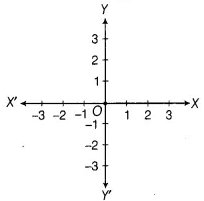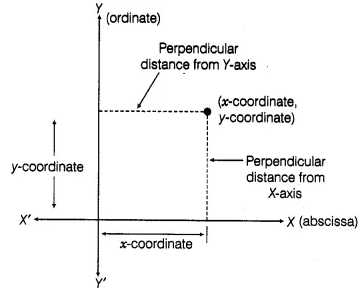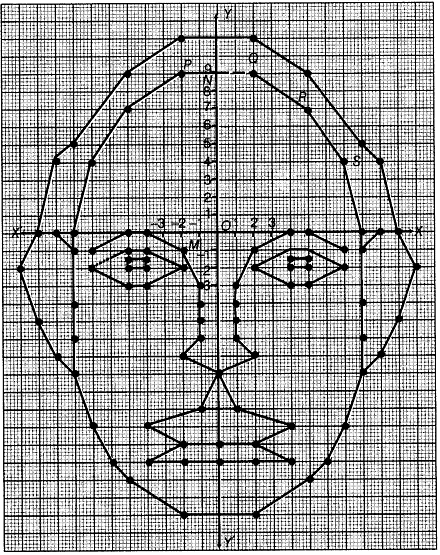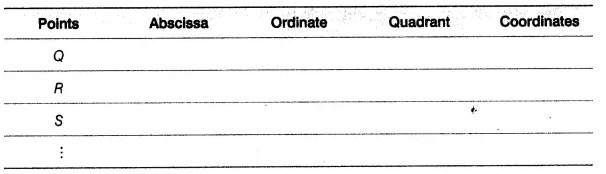# Lab Manual: Find the Values of Abscissae Notes | Study Lab Manuals for Class 9 - Class 9

## Class 9: Lab Manual: Find the Values of Abscissae Notes | Study Lab Manuals for Class 9 - Class 9

The document Lab Manual: Find the Values of Abscissae Notes | Study Lab Manuals for Class 9 - Class 9 is a part of the Class 9 Course Lab Manuals for Class 9.
All you need of Class 9 at this link: Class 9

Objective
To find the values of abscissae and ordinates of various ! points given in a cartesian plane.

Materials Required
Cardboard, White paper, Graph paper with various given points, Geometry box, Scissors, Glue

Prerequisite Knowledge
(i) Knowledge of cartesian coordinate system.
(ii) To locate/plot a point in cartesian plane.

Theory
1. Cartesian Coordinate System
The system used to describe the position of a point in a plane, is called cartesian system. In cartesian system, there are two perpendicular directed straight lines XX’ and YY’ which intersect at point 0, then line XX’ will be horizontal line and YY’ will be vertical line, shown x’ as alongside figure.

The point of intersection of these lines is called origin and it is denoted by O. In other words, the point from which distances are marked is called origin. The horizontal line XOX’ is called  X-axis and the vertical line YOY’ is called Y-axis. Directions OX Fig. 16.1Fig. 16.1

and OY are called the positive directions of X-axis and Y-axis, respectively and directions OX’ and OY’ are called the negative directions of X-axis and Y-axis, respectively.

Note:

• The angle between the horizontal and vertical axes is 90°.
• Any line perpendicular to the X-axis is parallel to the Y-axis.
• ny line perpendicular to the Y-axis is parallel to the X-axis.

2. Coordinates of a Point in Cartesian Plane
To locate a point in the cartesian plane, its perpendicular distances from X-axis and Y-axis are required, these distances are called coordinates of the point. Each point in cartesian plane has two coordinates; x-coordinate and y-coordinate.
(i) The x-coordinate of a point is the perpendicular distance from the Y-axis measured along the X-axis (positive, along the positive direction of the X-axis and negative, along the negative direction of X-axis). The x-coordinate is also called the abscissa.Fig. 16.2

(ii) The y-coordinate of a point is the perpendicular distance from the X-axis measured along the Y-axis (positive, along the positive direction of the Y-axis and negative, along the negative direction of Y-axis). The y-coordinate is also called the ordinate.
x-coordinate and y-coordinate taken together are called cartesian coordinates or coordinates of a point and denoted by (x, y). Here, the x-coordinate comes first and after this y-coordinate comes. (x, y) is called an order pair.
The order of x and y is important in (x, y). Position of (y, x) will be different from (x, y).

Procedure

• Take a cardboard of suitable size and paste a white paper on it.
• Now, paste the graph paper with various points drawn on it in the’ middle of white paper.
• Now, look at the graph paper and the points whose abscissae and ordinates are to be found.

Demonstration
If P is a point plotted on graph paper and we have to find the abscissa and ordinate of point P.
Draw perpendiculars PM and PN from P to X-axis and Y-axis, respectively.
PN = -2 units (distance from X-axis, i.e. x-coordinate or abscissa)
PM = 9 units (distance from Y-axis, i.e. y-coordinate or ordinate)
6As, point P lies in second quadrant.
So, the coordinates of P are (- 2,9).Note:
Care should be taken while reading the coordinates, otherwise the location of the object will differ.

ObservationFill the above table for different points on the given graph.

Result
The coordinates of the points plotted on the graph paper have been found.

Application
This activity is very useful in locating the position of a particular country/city or a place on map.

The document Lab Manual: Find the Values of Abscissae Notes | Study Lab Manuals for Class 9 - Class 9 is a part of the Class 9 Course Lab Manuals for Class 9.
All you need of Class 9 at this link: Class 9Use Code STAYHOME200 and get INR 200 additional OFF

## Lab Manuals for Class 9

108 docs

Track your progress, build streaks, highlight & save important lessons and more!

,

,

,

,

,

,

,

,

,

,

,

,

,

,

,

,

,

,

,

,

,

;